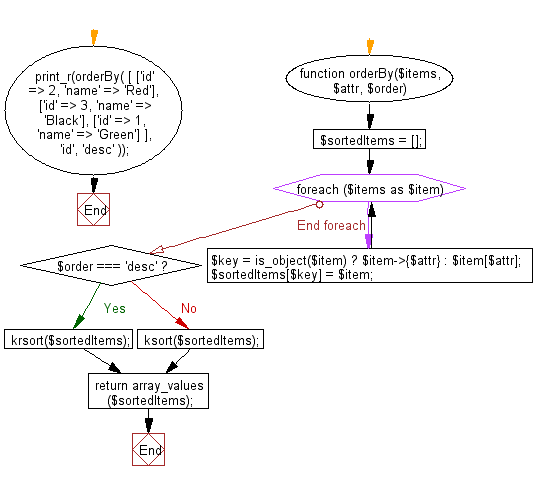﻿ PHP Exercise: Sort a collection of given arrays or objects by key - w3resource

# PHP Exercises: Sort a collection of given arrays or objects by key

## PHP: Exercise-93 with Solution

Write a PHP program to sort a collection of given arrays or objects by key.

Sample Solution: -

PHP Code:

``````<?php
//Licence: https://bit.ly/2CFA5XY

function orderBy(\$items, \$attr, \$order)
{
\$sortedItems = [];
foreach (\$items as \$item) {
\$key = is_object(\$item) ? \$item->{\$attr} : \$item[\$attr];
\$sortedItems[\$key] = \$item;
}
if (\$order === 'desc') {
krsort(\$sortedItems);
} else {
ksort(\$sortedItems);
}

return array_values(\$sortedItems);
}
print_r(orderBy(
[
['id' => 2, 'name' => 'Red'],
['id' => 3, 'name' => 'Black'],
['id' => 1, 'name' => 'Green']
],
'id',
'desc'
));
?>
```
```

Sample Output:

```Array
(
 => Array
(
[id] => 3
[name] => Black
)

 => Array
(
[id] => 2
[name] => Red
)

 => Array
(
[id] => 1
[name] => Green
)

)```

Flowchart:PHP Code Editor:

Have another way to solve this solution? Contribute your code (and comments) through Disqus.

What is the difficulty level of this exercise?

Test your Programming skills with w3resource's quiz.

﻿

## PHP: Tips of the Day

Mutates the original array to filter out the values specified

Example:

```<?php
function tips_pull(&\$items, ...\$params)
{
\$items = array_values(array_diff(\$items, \$params));
return \$items;
}

\$items = ['x', 'y', 'z', 'x', 'y', 'z'];
print_r(tips_pull(\$items, 'y', 'z'));
?>
```

Output:

```Array
(
 => x
 => x
)
```